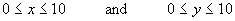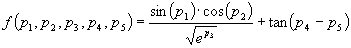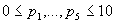Home
News
Author
Q&A
Tutorials
GEP Biblio
Contacts

Visit Gepsoft

Gene Expression Programming: Mathematical Modeling by an Artificial Intelligence

Maximum seeking with GEP

In this section we are going to seek the maximum of two different functions. The first, is the following, well-studied two-parameter function:(4.20a)

subject to the constraints:(4.20b)

For this function the maximum value is known, which, for simplicity, will be considered 18.5.

Although simple, this kind of functions with known solutions are very useful as they can be used to measure accurately the performance of different algorithms. For instance, the global minimum of the function (4.20) above could not be found by traditional minimum seeking algorithms such as the Nelder-Mead or the Broyden-Fletcher-Goldfarb-Shanno (BFGS) algorithms (Haupt and Haupt 1998). On the other hand, less conventional methods such as GAs or GEP have no problems at all in finding the global maximum to this function (Table 4.13). Indeed, considering 18.5 the maximum output for function (4.20), both GEP and a GA simulation with h = 0 (GEP-HZero) could find the exact parameters for which the function returns values equal to or greater than 18.5 in virtually all runs. As shown in Table 4.13, the GA simulation slightly outperforms GEP at this simple task. Taking into account the greater complexity of GEP, it is obviously advisable to use the simpler GA simulation to solve function optimization problems with one or two dimensions. However, the simple GA is known to converge prematurely, becoming frequently stuck in some local maximum. In those cases, the fine-tuning capabilities of gene expression programming become indispensable as the results obtained on our second function will show.

Table 4.13
Settings for the two-parameter function optimization problem using a GEP simulation of a GA in which the head is equal to zero (GEP-HZero) and gene expression programming (GEP).

 GEP-HZero GEP Number of runs 100 100 Number of generations 1000 1000 Population size 30 30 Function set -- + - * / Terminal set ? ? Random constants array length 1 10 Random constants range [0, 10] [-1, 1] Head length 0 6 Number of genes 2 2 Chromosome length 4 40 Mutation rate -- 0.044 One-point recombination rate -- 0.3 Two-point recombination rate -- 0.3 Gene recombination rate 0.8 0.1 IS transposition rate -- 0.1 IS elements length -- 1,2,3 RIS transposition rate -- 0.1 RIS elements length -- 1,2,3 Gene transposition rate 0.2 0.1 Random constants mutation rate 0.25 0.01 Dc specific transposition rate -- 0.1 Dc specific IS elements length -- 1,2,3 Average best-of-run output 18.5544 18.5351 Success rate 100% 93%

This function is the five-parameter function of section 4.1.2:(4.21a)

subject to the constraints:(4.21b)

The global maximum of this function is not known and, therefore, we won’t be able to compare the performance of the algorithms in terms of success rate; consequently, we will use the average best-of-run output for that purpose.

As shown in Table 4.14, in this problem, GEP significantly outperforms the simpler GA simulation. And the reason for this superiority consists exactly in the aforementioned tendency of the GA to converge prematurely on a local maximum. For instance, in the experiment shown in the first column of Table 4.14, the maximum value found by the GA is equal to 272243, and this value was again and again rediscovered by the GA (more precisely, in eight different runs). This kind of behavior is never observed in GEP. Curiously, in all the 100 runs, GEP never found the local maximum the GA was attracted to. Instead it found much higher peaks, including 293686, 342089, 346903, 1.90464 X 106, and 3.85419 X 106. The parameter values for the highest peak are:

 f (p1, p2, p3, p4, p5) = (1.5701, 0.00071129, 0, 1.58036, 0.00956748)

for which the function value at that point is:

 f (p1, p2, p3, p4, p5) = 3.85419 X 106.

Table 4.14
Settings for the five-parameter function optimization problem using a GEP simulation of a GA in which the head is equal to zero (GEP-HZero) and gene expression programming (GEP).

 GEP-HZero GEP Number of runs 100 100 Number of generations 1000 1000 Population size 30 30 Function set -- + - * / Terminal set ? ? Random constants array length 1 10 Random constants range [0, 10] [-1, 1] Head length 0 6 Number of genes 5 5 Chromosome length 10 100 Mutation rate -- 0.044 One-point recombination rate -- 0.3 Two-point recombination rate -- 0.3 Gene recombination rate 0.8 0.1 IS transposition rate -- 0.1 IS elements length -- 1,2,3 RIS transposition rate -- 0.1 RIS elements length -- 1,2,3 Gene transposition rate 0.2 0.1 Random constants mutation rate 0.25 0.01 Dc specific transposition rate -- 0.1 Dc specific IS elements length -- 1,2,3 Average best-of-run output 34691.2 98096.8

Home | Contents | Previous | Next